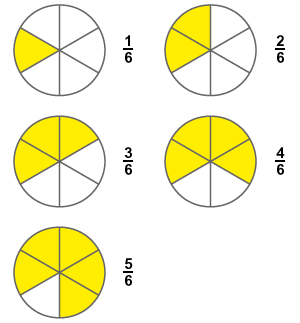# Proper fraction

Definition:
A fraction which contains the number of selected parts of a whole is less than the total number of parts of the same whole, is called proper fraction.

A whole quantity can be split into numerous equal parts and the selected portion from them is known as fraction. The number of selected parts from them is always less than the total number of parts of that whole quantity because it is possible and proper. Therefore, the fraction is known as proper fraction.

The value of every proper fraction is always less than 1 due to the ratio of less selected number of parts to total number of parts of the whole quantity.

## ExampleConsider a circle and divide it into six equal sectors.

1. Consider only one part from six parts of the one whole circle. The fraction of it is $\frac{1}{6}$.
2. Consider two parts from six parts of the one whole circle and the fraction of it is $\frac{2}{6}$.
3. Consider three parts from six parts of the one whole circle and the fraction of it is $\frac{3}{6}$.
4. Consider four parts from six parts of the one whole circle and the fraction of it is $\frac{4}{6}$.
5. Consider five parts from six parts of the one whole circle and the fraction of it is $\frac{5}{6}$.

Considering six parts does not create a fraction because it forms a whole circle. So, it is proper to select less number of parts than total parts. Hence, it is called as a proper fraction.

Therefore, $\frac{1}{6}$, $\frac{2}{6}$, $\frac{3}{6}$, $\frac{4}{6}$ and $\frac{5}{6}$ are rational numbers which represent five fractions. All five fractions have same denominator but different numerators. However, the values in numerators are less than the denominator.

Calculate, value of every fraction numerically.

$\frac{1}{6}=0.1667$

$\frac{2}{6}=0.3333$

$\frac{3}{6}=0.5$

$\frac{4}{6}=0.6667$

$\frac{5}{6}=0.8333$

The values of each fraction in decimal system does not exceed the 1. So, it is proved that the value of every proper fraction is always less than 1. It can be expressed in mathematical form.